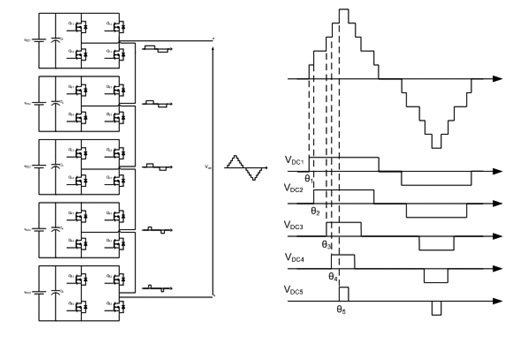# multilevel inverters introduction types advantages and applications

Multilevel inverters types applications, advantages and disadvantages all things have been explained in this article. In order to have a clear understanding of Multi-level inverters, one should have an explicit idea about inverters and its purpose in power electronics.

Power Electronics: The branch of electronics that deals with conversion and control of Electric power is called power electronics. Many of our appliances are designed to work with AC, but a power generator normally generates DC. So, if we want to run something like an AC-powered gadget from a DC car battery in a mobile home, we need a device that will convert DC to AC. Here comes the concept of Inverters.

Inverters: The device which converts DC voltage to AC voltage is called inverter. Output voltage should ideally be sinusoidal wave of variable magnitude and variable frequency. An inverter does the opposite job of Rectifier. Electronic inverters can be used to produce smoothly varying AC output from a DC input. Inverters are made up of capacitors and inductors which make the output current smooth as compared to switching square wave output you get with a basic inverter. Different types of inverters and their complete design can be checked by visiting following links

A best example to understand the use for inverters is in emergency power supplies, also called uninterruptible power supplies (UPS). In a typical UPS, when power is flowing normally, the batteries are charged by DC, which is produced from the AC power supply using a transformer and rectifier circuit. If the power fails, the batteries provide DC through an inverter in order to produce AC.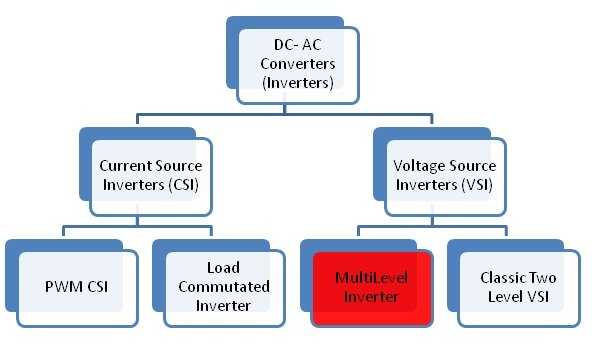Multilevel inverters are the preferred choice of industry for the application in high voltage and for high power application. Multilevel inverter technology has emerged recently as a very important alternative in the area of high-power medium-voltage energy control. This article presents the concept behind multi-level inverters, their types, pros and cons and the area of their practical applications.

### Why Multi-level Inverters?

The need of multilevel converter is to give a high output power from medium voltage source. The multi level inverter consists of several switches.

### How multilevel inverters Works?

The most common type of inverter which is used to generate AC voltage from DC Voltage is two level inverter. A two-level Inverter creates two different voltages for the load i.e. suppose we are providing V as an input to a two level inverter then it will provide + V/2 and – V/2 on output. In order to build an AC voltage, these two newly generated voltages are usually switched.Although this method of conversion of voltage is effective but it has some limitations as it causes disturbance in the output voltage.  Normally this method works but in some applications it creates problems specifically where high distortion in the output voltage is not required.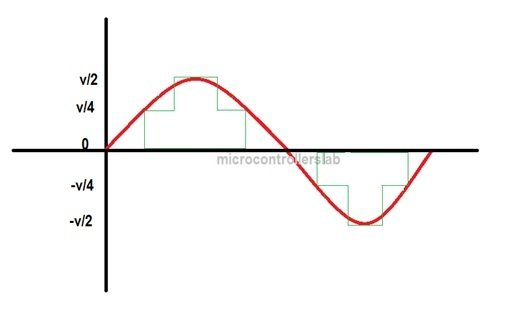The concept of multilevel Inverter (MLI) is kind of modification of two-level inverter. In multilevel inverters we don’t deal with the two level voltages instead in order to create a smoother stepped output waveform, more than two voltage levels are combined together. Smoothness of the waveform is directly proportional to the voltage levels, as we increase the voltage level, the waveform becomes more smoother but the complexity will be increased.

### Types of Multilevel Inverters: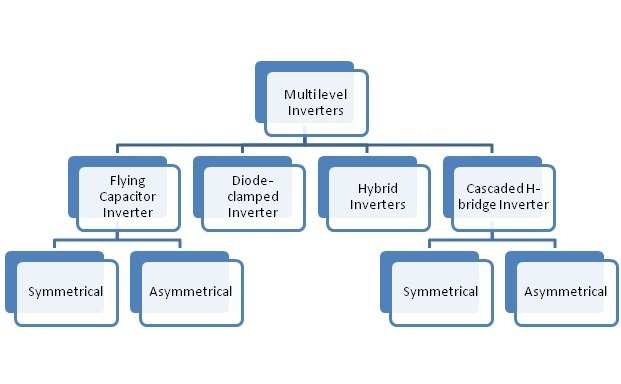• ### Diode Clamped Multilevel Inverter

This type of inverter uses diodes and gives different voltage levels to the capacitor banks connected in series. The benefit of using diode is to reduce stress on other electrical devices because it gives a limited amount of voltage. But there is a drawback of this topology that the maximum voltage which we can get from it cannot be more than half of input voltage (DC voltage). But this problem can be solved by increasing the number of capacitors, switches and diodes. This type of inverters provides the high efficiency and it is a simple method of the back to back power transfer systems.Example: 5- Level diode clamped multilevel inverter, 9- level diode clamped multilevel inverter.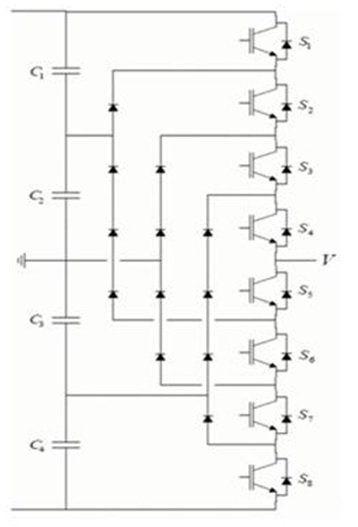• ### Flying Capacitors Multilevel Inverter

The main idea of this topology is the use of capacitors. The capacitors transfer the limited amount of voltage to electrical devices. In this inverter switching states are like in the diode clamped inverter. Clamping diodes are not required in this type of inverters. Likewise, the maximum voltage which we can get from it cannot be more than half of input voltage (DC voltage). It can control both the active and reactive power flow. But due to the high frequency switching, switching losses will takes place.Example: 5-level flying capacitors multilevel inverter, 9-level flying capacitors multilevel inverter.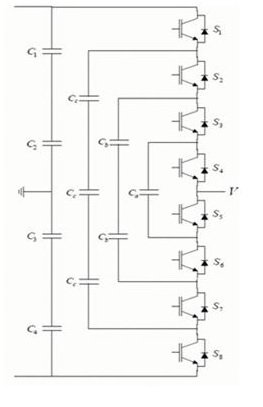• This inverter is same like that diode clamped multi inverter
• In this inverter only switches and capacitors are used.
• ### Cascaded H-bridge Multilevel inverter

The Cascaded H-bridge multilevel inverter uses capacitor and switches.  It requires less number of components in each level as compared to previous described types. This topology consists of series of power conversion cells and power can be easily scaled.The combination of capacitors and switches pair is called an H-bridge.It consists of H-bride cells and each cell can provide three different voltages like zero, positive DC and negative DC voltages.Example: 5-H-Bridge, 9-H-Bridge Multilevel inverter